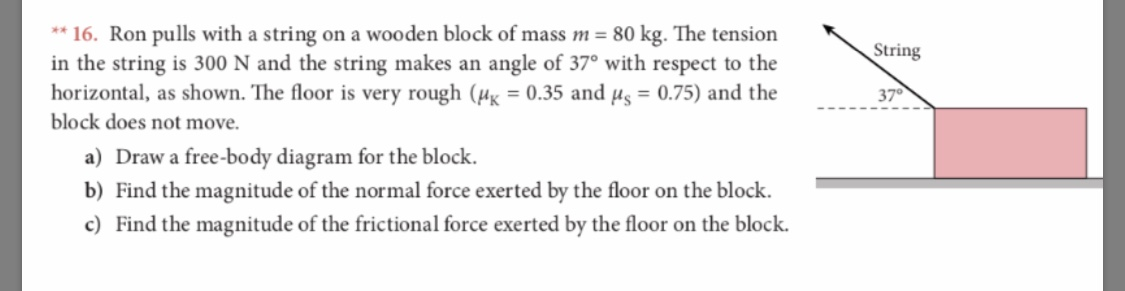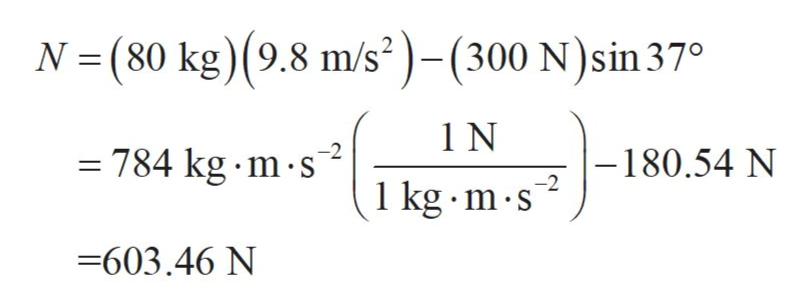*16. Ron pulls with a string on a wooden blo ck of mass m = 80 kg. The tensionin the string is 300 N and the string makes anhorizontal, as shown. The floor is very rough (H = 0.35 and ug = 0.75) and theblock does not moveStringangle of 37° with respect to the37a) Draw a free-body diagram for the blockb) Find the magnitude of the normal force exerted by the floor on the blockc) Find the magnitude of the frictional force exerted by the floor on the block.

Questionhelp_outlineImage Transcriptionclose*16. Ron pulls with a string on a wooden blo ck of mass m = 80 kg. The tension in the string is 300 N and the string makes an horizontal, as shown. The floor is very rough (H = 0.35 and ug = 0.75) and the block does not move String angle of 37° with respect to the 37 a) Draw a free-body diagram for the block b) Find the magnitude of the normal force exerted by the floor on the block c) Find the magnitude of the frictional force exerted by the floor on the block. fullscreen
Step 1

(a)

Consider the FBD of the block as shown.

Step 2

Here, N is the normal reaction force that acts on the block and f is the friction’s force.

(b)

Since the block is at rest, the net horizontal and vertical forces that act on the block must be zero each.

Equate the net vertical forces to zero. Take the forces along the upward direction to be positive.

Step 3

Substitute 300 N for T and 80 ...help_outlineImage TranscriptioncloseN (80 kg) (9.8 m/s2)-(300 N)sin 37° 1 N = 784 kg m s -180.54 N -2 1 kg m s -603.46 N fullscreen

Want to see the full answer?

See Solution

Want to see this answer and more?

Our solutions are written by experts, many with advanced degrees, and available 24/7

See Solution
Tagged in

Newtons Laws of Motion